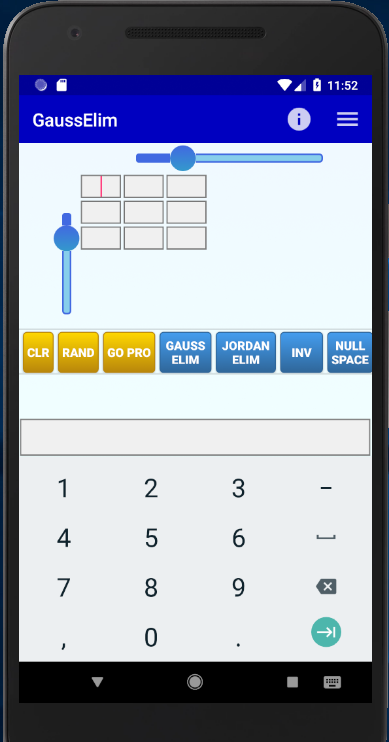# latest news

## 04.04.2014

New paper accepted in CIP 2014

# GaussElim

An Android application to perform Gauss Elimination

Author: Pantelis BouboulisGaussElim is a simple application that applies the Gaussian Elimination process to a given matrix. You can find the application on PlayStore (there is also a GaussElim Pro version without any ads). You can set the matrix dimensions using the scrollbars and then you can edit the matrix elements by typing in each cell (the cells become active/inactive once you move the respective scrollbar). You can move to another cell either by pressing the NEXT key on the soft keyboard, or by tapping the desired cell. After you have entered the entries of the desired matrix, you can press one of the available buttons (described below):

CLR: Clears the contents of the matrix.

Gauss Elim: Applies the Gauss elimination process to the given matrix. The result is an unreduced Row-Echelon matrix.

Jordan Elim: Applies the Gauss-Jordan elimination process to the given matrix. The result is a reduced Row-Echelon matrix.

INV: Applies the Gauss-Jordan elimination process to find (if possible) the inverse of the given matrix.

Null Space: Finds the Null space of the given matrix by applying the Gauss-Jordan Elimination Process.

Col Space: Finds the column space of the given matrix by applying the Gauss Jordan elimination process to the transpose matrix.

Row Space: Finds the row space of the given matrix by applying the Gauss-Jordan elimination process. Note that the row Space can be found also by using either the Gauss Elim button or the Jordan button.

Gram Schmidt: Orthogonalizes the (column) vectors of the matrix following the Gram-Schmidt method.

Gram Schmidt+: Orthonormalizes the (column) vectors of the matrix following the Gram-Schmidt method.